##### The Japanese Bonsai specialist
Direct order Contact Help / Services Newsletter# Hosta siberian tiger

Hosta siberian tiger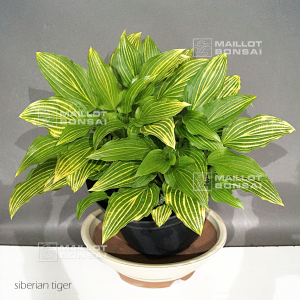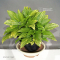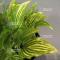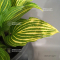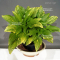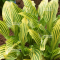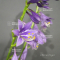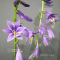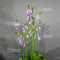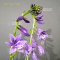ref. : 11648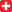14,00

Available quantity : 3Order

###### Description

Rare en cultivation in Europe .
Coming from a hybridization Hosta 'Mito no hana'.

Dimensions of the plant :  35/40 cm high by about 30 cm wide.

Dimensions of the leaves : about 30 cm long.

Oval green leaves covered by yellow stripes (mostly during spring) that fade slowly with the arrival of summer. A characteristic that gives this hosta a beautiful aspect.

Mauve colour flowering from June to july.

Big rhizome delivered in a 1 liter plastic pot.

#hosta 4.5 #dimensions 3 #siberian 2.8 #hostas 2.6 #leaves 2.6 #about 2.5 #tiger 2.5 #characteristic 2.4 #that 2.4 #from 2.4

###### Technical description
 height 30-50 cm

Formule
(( ROUND((CHAR_LENGTH(b.article_nom)-CHAR_LENGTH(REPLACE(b.article_nom, 'hosta', '')))/LENGTH('hosta')) + ROUND((CHAR_LENGTH(b.article_description)-CHAR_LENGTH(REPLACE(b.article_description, 'hosta', '')))/LENGTH('hosta')) ) * 4.5) + (( ROUND((CHAR_LENGTH(b.article_nom)-CHAR_LENGTH(REPLACE(b.article_nom, 'dimensions', '')))/LENGTH('dimensions')) + ROUND((CHAR_LENGTH(b.article_description)-CHAR_LENGTH(REPLACE(b.article_description, 'dimensions', '')))/LENGTH('dimensions')) ) * 3) + (( ROUND((CHAR_LENGTH(b.article_nom)-CHAR_LENGTH(REPLACE(b.article_nom, 'siberian', '')))/LENGTH('siberian')) + ROUND((CHAR_LENGTH(b.article_description)-CHAR_LENGTH(REPLACE(b.article_description, 'siberian', '')))/LENGTH('siberian')) ) * 2.8) + (( ROUND((CHAR_LENGTH(b.article_nom)-CHAR_LENGTH(REPLACE(b.article_nom, 'leaves', '')))/LENGTH('leaves')) + ROUND((CHAR_LENGTH(b.article_description)-CHAR_LENGTH(REPLACE(b.article_description, 'leaves', '')))/LENGTH('leaves')) ) * 2.6) + (( ROUND((CHAR_LENGTH(b.article_nom)-CHAR_LENGTH(REPLACE(b.article_nom, 'about', '')))/LENGTH('about')) + ROUND((CHAR_LENGTH(b.article_description)-CHAR_LENGTH(REPLACE(b.article_description, 'about', '')))/LENGTH('about')) ) * 2.5) + (( ROUND((CHAR_LENGTH(b.article_nom)-CHAR_LENGTH(REPLACE(b.article_nom, 'tiger', '')))/LENGTH('tiger')) + ROUND((CHAR_LENGTH(b.article_description)-CHAR_LENGTH(REPLACE(b.article_description, 'tiger', '')))/LENGTH('tiger')) ) * 2.5) + (( ROUND((CHAR_LENGTH(b.article_nom)-CHAR_LENGTH(REPLACE(b.article_nom, 'that', '')))/LENGTH('that')) + ROUND((CHAR_LENGTH(b.article_description)-CHAR_LENGTH(REPLACE(b.article_description, 'that', '')))/LENGTH('that')) ) * 2.4) + (( ROUND((CHAR_LENGTH(b.article_nom)-CHAR_LENGTH(REPLACE(b.article_nom, 'from', '')))/LENGTH('from')) + ROUND((CHAR_LENGTH(b.article_description)-CHAR_LENGTH(REPLACE(b.article_description, 'from', '')))/LENGTH('from')) ) * 2.4) + (( ROUND((CHAR_LENGTH(b.article_nom)-CHAR_LENGTH(REPLACE(b.article_nom, 'characteristic', '')))/LENGTH('characteristic')) + ROUND((CHAR_LENGTH(b.article_description)-CHAR_LENGTH(REPLACE(b.article_description, 'characteristic', '')))/LENGTH('characteristic')) ) * 2.4) + (( ROUND((CHAR_LENGTH(b.article_nom)-CHAR_LENGTH(REPLACE(b.article_nom, 'hybridization', '')))/LENGTH('hybridization')) + ROUND((CHAR_LENGTH(b.article_description)-CHAR_LENGTH(REPLACE(b.article_description, 'hybridization', '')))/LENGTH('hybridization')) ) * 2.3)

## Secure payment## Delivery

Our logistic partners :04 74 55 23 48
Pépinière MAILLOT-BONSAÏ
Le Bois Frazy
01990 RELEVANT - FRANCE
on appointment